## Example Questions

### Example Question #7 : Rational Expressions

Which of the following is equivalent to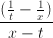? Assume that denominators are always nonzero.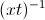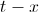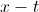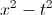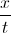Explanation:

We will need to simplify the expression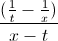. We can think of this as a large fraction with a numerator of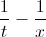and a denominator of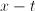.

In order to simplify the numerator, we will need to combine the two fractions. When adding or subtracting fractions, we must have a common denominator.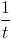has a denominator of, and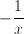has a denominator of. The least common denominator that these two fractions have in common is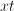. Thus, we are going to write equivalent fractions with denominators of.

In order to convert the fraction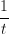to a denominator with, we will need to multiply the top and bottom by.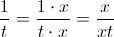Similarly, we will multiply the top and bottom ofby.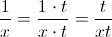We can now rewriteas follows:=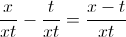Let's go back to the original fraction. We will now rewrite the numerator:=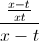To simplify this further, we can think ofas the same as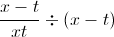. When we divide a fraction by another quantity, this is the same as multiplying the fraction by the reciprocal of that quantity. In other words,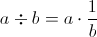.=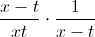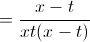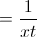Lastly, we will use the property of exponents which states that, in general,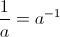.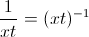The answer is.